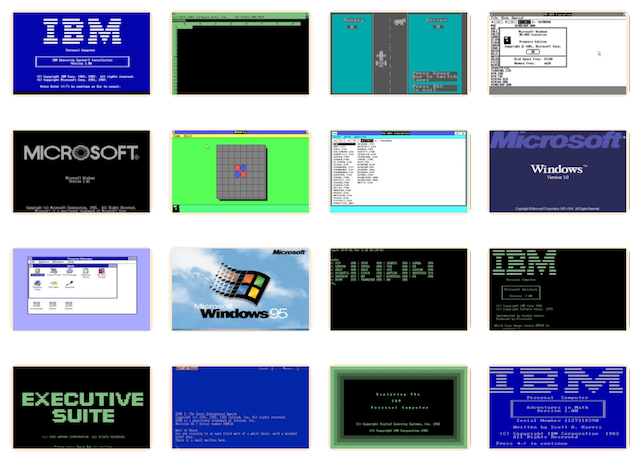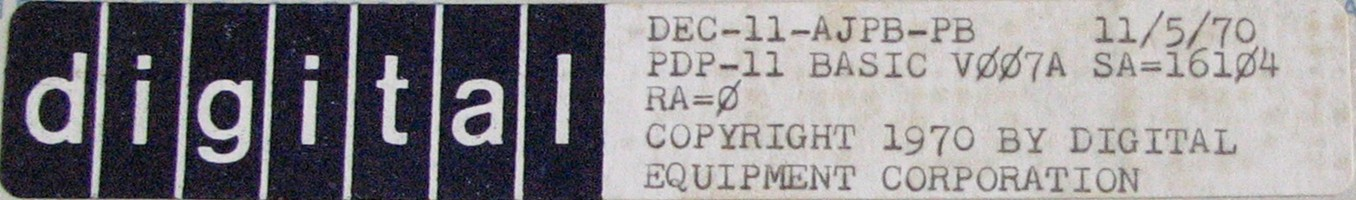# PCjs Machines

Home of the original IBM PC emulator for browsers.## DEC PDP-11 BASICAccording to the PDP-11/20 Handbook (1971), p. 160, notable features of PDP-11 BASIC included:

• Use of BASIC statements in immediate mode (no line number)
• Ability to use any BASIC command (RUN, LIST, etc.) in deferred mode (with a line number)
• Recursive subroutine calls
• Multiple statements on a single line
• Array names of a letter followed by a number
• User programs can be halted (with CTRL/P) without clearing variables; PRINT can then be used to examine values
• Ability to call assembly language functions

PDP-11 BASIC has been loaded into the PDP-11/20 below.

[PCjs Machine "test1120"]

Waiting for machine "test1120" to load....

We have archived the following DEC PDP-11 BASIC resources:

Third-party resources include:

## Debugging Notes

### PDPjs Debugger vs. SIMH

When I first tried to run BASIC in a PDPjs machine, it crashed almost immediately. It was attempting to use memory beyond the 16Kb of installed RAM. After a bit of poking around, I found BASIC’s memory sizing code here:

``````016142: 012701 160000          MOV   #160000,R1
016146: 022626                 CMP   (SP)+,(SP)+
016150: 014111                 MOV   -(R1),@R1
``````

The code sets R1 to highest possible RAM address and starts scanning backwards for the first valid memory location. However, the scanning process wasn’t clear to me at first glance, and the `CMP (SP)+,(SP)+` was a bit of a head-scratcher, so I decided to do an instruction-by-instruction comparison with SIMH.

After cloning the SIMH project and building the pdp11 binary, I created a pdp11.ini text file that contained:

``````ECHO Configuring PDP-11/20 with 16Kb of RAM...
SET CPU 11/20
SET CPU 16K

; Throttle SIMH to limit CPU usage, heat and fan noise
SET THROTTLE 5%

; Disable devices that we don't need
SET HK DISABLE
; SET RHA DISABLE
SET DZ DISABLE
SET RL DISABLE
SET RX DISABLE
SET RP DISABLE
SET RQ DISABLE
SET TM DISABLE
SET TQ DISABLE
SET RK DISABLE

; Enable the high-speed paper tape reader
SET PTR ENABLE
; SET PTP ENABLE

ECHO Setting breakpoint at 016142...
break -e 016142
``````

along with a tr text file that contained:

``````step
examine r0,r1,r2,r3,r4,r5,sp,psw
``````

Then I ran pdp11 and typed “g” to start the machine:

``````PDP-11 simulator V4.0-0 Beta        git commit id: 592deb8f
Configuring PDP-11/20 with 16Kb of RAM...
Disabling XQ
Setting breakpoint at 016142...
sim> g

Breakpoint, PC: 016142 (MOV #160000,R1)
sim> do tr

Step expired, PC: 016146 (CMP (SP)+,(SP)+)
R0:	004106
R1:	160000
R2:	000000
R3:	000000
R4:	000000
R5:	000000
SP:	013654
PSW:	000010	CM=K PM=K RS0 FPD0 IPL=0 TBIT0 N1 Z0 V0 C0
sim> do tr

Step expired, PC: 016150 (MOV -(R1),(R1))
R0:	004106
R1:	160000
R2:	000000
R3:	000000
R4:	000000
R5:	000000
SP:	013660
PSW:	000011	CM=K PM=K RS0 FPD0 IPL=0 TBIT0 N1 Z0 V0 C1
sim> do tr

Step expired, PC: 016152 (SUB #302,R1)
R0:	004106
R1:	157776
R2:	000000
R3:	000000
R4:	000000
R5:	000000
SP:	013660
PSW:	000011	CM=K PM=K RS0 FPD0 IPL=0 TBIT0 N1 Z0 V0 C1
sim> do tr

Step expired, PC: 016150 (MOV -(R1),(R1))
R0:	004106
R1:	157776
R2:	000000
R3:	000000
R4:	000000
R5:	000000
SP:	013660
PSW:	000000	CM=K PM=K RS0 FPD0 IPL=0 TBIT0 N0 Z0 V0 C0
``````

After typing several “do tr” commands, I was surprised to see SIMH execution continually returning to this instruction:

``````Step expired, PC: 016150 (MOV -(R1),(R1))
``````

until I remembered that when the PDP-11 accesses an invalid address, it’s supposed to trap to vector 000004, and that BASIC must have modified vector 000004 to jump into the middle of this code.

This code fragment was simply marching down the address space until it reached an address that didn’t trap. The odd-looking `CMP (SP)+,(SP)+` instruction was throwing away the PC and PSW that each trap pushed onto the stack, by effectively adding 4 to SP.

The problem with PDPjs was that it wasn’t generating a trap to vector 000004 when an invalid address was accessed. After fixing that, I verified with the PDPjs Debugger that the memory sizing code was working properly:

``````PDPjs v1.30.1
Portions adapted from the PDP-11/70 Emulator v1.4 by Paul Nankervis <paulnank@hotmail.com>
bus: 00016Kb RAM at 000000
bus: 00008Kb H/W at 160000
cpu: model 1120
Type ? for help with PDP11 Debugger commands
R0=000000 R1=000000 R2=000000 R3=000000 R4=000000 R5=000000
SP=000000 PC=016104 PS=000013 T0 N1 Z0 V1 C1
016104: 016706 175602          MOV   013712,SP
>> bp 016142
bp 016142 set
>> g
running

bp 016142 hit
stopped (821 instructions, 7443 cycles, 23 ms, 323609 hz)
R0=004106 R1=000000 R2=000000 R3=000000 R4=000000 R5=000000
SP=013654 PC=016142 PS=000000 T0 N0 Z0 V0 C0
016142: 012701 160000          MOV   #160000,R1
>> u
016146: 022626                 CMP   (SP)+,(SP)+
016150: 014111                 MOV   -(R1),@R1
016152: 162701 000302          SUB   #302,R1
016156: 010167 001300          MOV   R1,017462
016162: 012700 013540          MOV   #13540,R0
016166: 104552                 TRAP  152
016170: 122702 000114          CMPB  #114,R2
016174: 001433                 BEQ   016264
>> dw 4 l2
000004  016146  000000
>> tr
R0=004106 R1=160000 R2=000000 R3=000000 R4=000000 R5=000000
SP=013654 PC=016146 PS=000010 T0 N1 Z0 V0 C0
016146: 022626                 CMP   (SP)+,(SP)+            ;cycles=7
>> tr
R0=004106 R1=160000 R2=000000 R3=000000 R4=000000 R5=000000
SP=013660 PC=016150 PS=000011 T0 N1 Z0 V0 C1
016150: 014111                 MOV   -(R1),@R1              ;cycles=11
>> tr
trapped to 004 (157776)
R0=004106 R1=157776 R2=000000 R3=000000 R4=000000 R5=000000
SP=013654 PC=016146 PS=000000 T0 N0 Z0 V0 C0
016146: 022626                 CMP   (SP)+,(SP)+            ;cycles=0
>> tr
R0=004106 R1=157776 R2=000000 R3=000000 R4=000000 R5=000000
SP=013660 PC=016150 PS=000000 T0 N0 Z0 V0 C0
016150: 014111                 MOV   -(R1),@R1              ;cycles=11
>> tr
trapped to 004 (157774)
R0=004106 R1=157774 R2=000000 R3=000000 R4=000000 R5=000000
SP=013654 PC=016146 PS=000000 T0 N0 Z0 V0 C0
016146: 022626                 CMP   (SP)+,(SP)+            ;cycles=0
>> tr
R0=004106 R1=157774 R2=000000 R3=000000 R4=000000 R5=000000
SP=013660 PC=016150 PS=000000 T0 N0 Z0 V0 C0
016150: 014111                 MOV   -(R1),@R1              ;cycles=11
>> g
running
PDP-11 BASIC, VERSION 007A
*O
``````

I like to think that the PDPjs Debugger (and in fact, all PCjs Debuggers) is considerably simpler to use, and more informative, too. For example, when you’re single-stepping (“t” or “tr”), the Debugger will alert you if the previous instruction triggered a trap.

There are also a variety of diagnostic “bus” and “memory” messages that can be enabled, if you want to see more detail about each instruction’s operation; see the Debugger’s “m” command for details.

### TRAP Handling

One of the first things I noticed when debugging PDP-11 BASIC was its heavy reliance on TRAP instructions. For example, `TRAP 000` is used to output the character in R2 to the terminal. Let’s take a closer look at how its TRAP handler works.

Since the trap vector for TRAP instructions is fixed at address 000034, we need to dump the contents of that vector:

``````>> dw 034 l2
000034  000100  000000
``````

This tells us that the BASIC TRAP handler is at 000100:

``````>> u 000100
000100: 011666 000002          MOV   @SP,2(SP)
000104: 162716 000002          SUB   #2,@SP
000110: 013646                 MOV   @(SP)+,-(SP)
000112: 006216                 ASR   @SP
000114: 103404                 BCS   000126
000116: 006316                 ASL   @SP
000124: 013607                 MOV   @(SP)+,PC
``````

When the code starts, the TRAP instruction has already pushed two words onto the stack:

``````0(SP):  previous PC
2(SP):  previous PSW
``````

The first instruction, `MOV @SP,2(SP)`, copies the previous PC onto the previous PSW, which is where we’ll eventually want previous PC, so that the handler can eventually return with a simple `RTS PC`.

The next instruction, `SUB #2,@SP`, subtracts 2 from the original previous PC, so that it now points to the TRAP instruction.

Then `@(SP)+,-(SP)` fetches the TRAP instruction while also “popping” the original previous PC and then “pushing” TRAP instruction onto the stack, overwriting the original previous PC.

The next few instructions shift the TRAP right to see if bit 0 is set, and if it is not, then the TRAP instruction is restored by shifting it left again, and then a large offset is added to it, transforming the TRAP instruction (which is now known to be an even value) into a jump table index.

The final instruction, `MOV @(SP)+,PC`, moves the address at the jump table index into PC, while also removing the TRAP instruction from the stack, leaving only the previous PC on the stack, so that when the TRAP handler is done, it can execute `RTS PC` to return to the caller.